# Food Technology: Exponential Growth of Bacteria

A study is done in which the number of bacteria in a liquid culture is monitored over a period of several hours. Ten observations were made: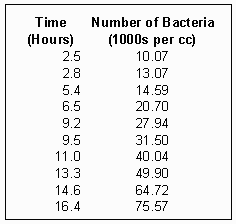Two questions need to be answered:

1. Is this data consistent with an exponential growth model: N = N0ekt, where
• N0 = the number of bacteria present initially (at t = 0)
• k = a growth rate constant
• N = the number of bacteria present at a given time t,
• and e is the fundamental mathematical constant forming the base for natural logarithms.
2. If the answer to the first question is "yes", then what are the values of N0, k, and t2 (the so-called doubling time) for this bacterial system?

The experimental measurements listed above indicate the number of bacteria are growing with time. If we plot the observed values of N (vertically) against the elapsed time, t, in hours, we get the graph: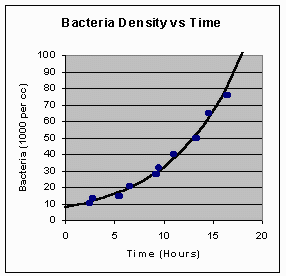You can draw a smooth curve through the experimental points as shown in this figure, and the points all seem to lie relatively close to this curve. Further, this curve looks quite a bit like the graph of an exponential function, but from what we see, it might just as well be part of a parabola or of one of a number of other curves that result when various algebraic forms are graphed. This graph suggests that the answer to the first question may be "yes", but we would usually want stronger evidence than this to draw a definite conclusion. The trouble here is that it is difficult to distinguish precisely and reliably between the curves are the graphs of quite different mathematical functions.

There is, of course, one curve that is easily recognized by everyone - the straight line! In this situation, the logarithmic function can be used to rewrite the exponential growth formula into a form that will allow us to create a plot that does give a straight line when data is the result of an exponential growth (or decay) process. Start with the exponential growth formula:

N = N0 ekt

Since this involves an exponential with base e, it is "natural" to use natural logarithms - those based on the number e. Using the more common notation ‘ln’ for ‘loge’, we simply take the logarithm of both sides of the previous equation:

ln N = ln(N0 ekt)

= ln N0 + ln(ekt)

= ln N0 + kt ln e

= ln N0 + kt

Here, we have used two of the three basic properties of logarithms to manipulate the right-hand side, namely that

log (AB) = log A + log B

log (Am) = m log A

Further, in the last step we took advantage of the fact that by choosing to work with natural logarithms, ln e = logee = 1. Thus, the exponential growth formula can be written in the logarithmic form

ln N = kt + ln N0

If the exponential growth formula is correct and we were to plot ln N along the vertical axis of our graph (rather than N itself), and t along the horizontal axis, then the equation of the plot we are preparing will have the generic form

vertical quantity = constant x horizontal quantity + another constant

But this is just the equation of a straight line. The plot that results using the data given above is: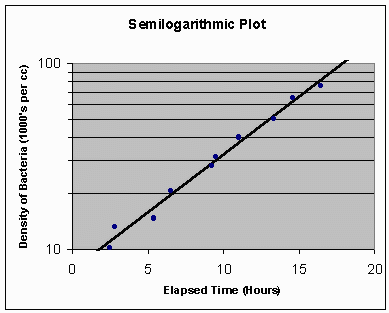Although the values written on the vertical axis are values of N, this graph is ruled vertically so that the vertical distances are actually proportional to ln N. Since one scale is logarithmic (the vertical one) whereas the other graph scale, the horizontal one, is the ordinary uniform spacing type, this is called a ‘semilogarithmic plot’.

At any rate, the figure indicates that the points are scattered about a straight line, the scatter due to small random errors in measurement. Since the plot of ln N vs t gives a straight line, we have confirmed that

ln N = kt + ln N0 (equation *)

is a valid formula for this data, and therefore, so is its equivalent, N = N0 ekt, valid for this data. This ends our answer to the first question posed.

It is now easy to answer the second question posed. Since we have concluded that (*) correctly describes the straight line graph in the semilogarithmic plot, we notice that the constant k is just the slope of this line. To calculate the slope, we first read the coordinates of two points on the line. Working with some care, for instance, we can determine that two such points are:

t = 1.80, N = 10

t = 17.95, N = 100

(for this sort of work, it’s important to pick your points as widely spaced as possible). These give us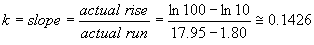Notice that the ‘actual rise’ has to be the actual vertical distance between the two points, and because the vertical scale is ruled off in proportion to ln N, this vertical distance will be the difference between the natural logarithms of the N values for the two points.

It would be easy to read the value of N0 directly off of the graph if we could see the point where the straight line crosses the t = 0 position. This is because when t = 0, we of course have simply

N = N0

and so the value of N0 could be read directly off of the graph. Unfortunately, because of the way we cropped our semilogarithmic plot, this is not possible in this example. Instead, we can substitute the coordinates of one of the points we’ve read from the graph into formula (*) above to get

ln 100 = (0.1426)(17.95) + ln N0

to give

ln N0 = ln 100 - (0.1426)(17.95) ≅ 2.04595

Thus,

N0 ≅ e2.04595 ≅ 7.737.

Finally, to compute the doubling time, t2, for this system, the time required for the number of bacteria to double, we use the formula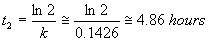(The units on t2 come about because if you trace the work above carefully, you find that k has units of hours-1. Also, you can derive the formula above from the original formula describing exponential growth by substituting N = 2N0 and solving for t.)

In summary then, we have obtained the following results:

1. we have confirmed that the data given at the beginning of the problem is indeed consistent with an exponential growth model.
2. based on that we have been able to estimate the constants in the exponential growth formula for this system, to get

N ≅ 7.737 e0.1426t

3. based on these results, we have determined that the number of bacteria will double approximately every 4.86 hours.Written by David Sabo, October 7, 1997